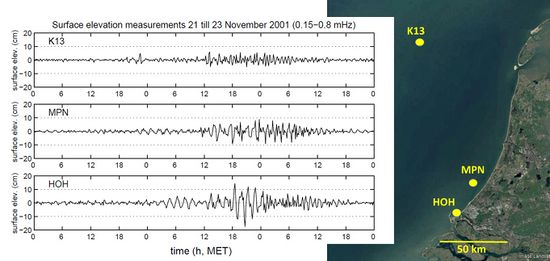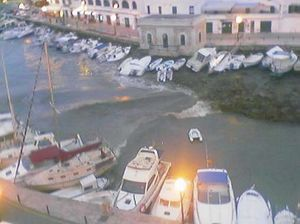# Proudman resonance and meteo tsunamis

## Generation of meteo tsunamisFig. 1. Amplification of meteo-induced wave motion travelling southward across the Southern North Sea toward the coast of Holland. From De Jong (2004) with permission.

Local atmospheric pressure disturbances above open water generate wave motions at the water surface. The periods of these meteo-induced waves are typically in the range of a few minutes to a few hours, i.e. intermediate between wind waves and tidal waves. The amplitude of these waves is generally small (typically in the cm range) compared to wind waves and tidal waves. However, in some cases they can be strongly amplified. This occurs when the pressure perturbation advances at a speed comparable to the propagation speed (celerity) of the surface waves in the water body. This amplification is known as Proudman resonance, after the scientist who first demonstrated this phenomenon. When meteo-induced water waves are strongly amplified they are often called meteo tsunamis, because their period is in the same range where tsunamis caused by other phenomena occur, such as submarine earthquakes and landslides – see the article Tsunami. Various atmospheric processes can generate meteo tsunamis, for example frontal passages, gales, squalls, storms, orographic influence and tornados. An example of a meteo tsunami is shown in Fig. 1.

## Proudman resonance

Whether the propagation speed $V$ of an atmospheric pressure disturbance is comparable to the celerity $c$ of surface waves depends on the depth of the water body, which can be approximated by $c=\sqrt{gh}$, where $h$ is the local water depth and $g \approx 9.8 \, m^2 s^{-1}$ the gravitational acceleration. In deep ocean water the wave propagation celerity is always much larger (typically in the order of 200 m/s) than the speed of atmospheric pressure disturbances (typically in the order of 10-30 m/s). However, on the continental shelf (depths between 50 and 500 m), the speeds can be comparable and Proudman resonance can occur. The mathematical derivation by Proudman is presented in the appendix.

## Harbor seichesFig. 2. Harbor seiche in Ciutadella Inlet (Menorca Island, Spain) generated by a meteo tsunami on 15 June 1996. Image from Montserrat et al. (2006), Creative Commons Licence.

Proudman resonance can amplify the initial meteo-induced water wave by a factor 5-10 if the propagation speeds of the atmospheric disturbance and the water wave are about the same. Even in this case the amplitude of the meteo tsunami will not exceed a few decimeters. Waves of this amplitude generally do not cause great harm. However, further amplification can occur if the wave period is close to the resonance period of coastal topographic features, such as semi-enclosed bays and basins. Many harbors resonate at wave periods in the same range as the periods of meteo tsunamis, on the order of one minute period for small harbors and on the order of one hour for large harbors (the basic resonance period of an elongate harbor of length $L$ and depth $h$ is given by $4L/\sqrt{gh}$). If the harbor resonance period and the wave period match, a further wave amplification with a factor 5-10 is possible. Resonant harbor waves are called seiches. For a more detailed discussion of this phenomenon, the reader is referred to the article Harbor resonance. Harbor seiches can cause considerable damage to berthed vessels and harbor infrastructure (Fig. 2).

## Appendix: A simple mathematical model of Proudman resonance

The simple model, first described by Proudman, considers the following idealised situation:

• Continental shelf of uniform depth ,
• The influence of friction, tide and earth's rotation are neglected.

The pressure disturbance is assumed uniform in $y$-direction and moves without deformation in $x$-direction with velocity $V$. The disturbance is then given by $p=-g \rho f(x-Vt)$, where $\rho$ is water density. The shallow-water equations of motion read

$\Large\frac{\partial u}{\partial t}\normalsize + g \Large\frac{\partial \zeta}{\partial x}\normalsize = - \Large\frac{1}{\rho}\frac{\partial p}{\partial x}\normalsize , \quad h\Large\frac{\partial u}{\partial x}\normalsize + \Large\frac{\partial \zeta}{\partial t}\normalsize = 0$.

Elimination of $u$ gives the equation $\Large\frac{\partial^2 \zeta}{\partial t^2}\normalsize -gh\Large\frac{\partial^2 \zeta}{\partial x^2}\normalsize = -gh\Large\frac{\partial^2 }{\partial x^2} \normalsize f(x-Vt)$.

The general solution reads

$\zeta(x,t)=\zeta^{(1)}(x-ct)+ \zeta^{(2)}(x+ct)+ \zeta^{(3)}(x-Vt), \qquad \zeta^{(3)}=\Large\frac{f}{1-(V/c)^2}\normalsize , \quad c=\sqrt{gh}$.

The functions $\zeta^{(1)}$ and $\zeta^{(2)}$ are determined by the initial conditions $\zeta=0, u=0$ at $t=0$. Evaluation of these conditions yields

$\zeta(x,t)=\Large\frac{1}{1-(V/c)^2}\normalsize \Big[ f(x-Vt) - \Large\frac{1}{2}\normalsize (1+\Large\frac{V}{c})\normalsize f(x-ct) - \Large\frac{1}{2}\normalsize (1-\Large\frac{V}{c})\normalsize f(x+ct) \Big]$.

If the propagation speed of the disturbance is close to the wave celerity, $V =c+dc$, the expression of $\zeta$ can be approximated by

$\zeta(x,t) \approx -\Large\frac{Vt}{2}\frac{\partial}{\partial x} \normalsize f(x-VT), \qquad x \le Vt$.

In this case we have Proudman resonance: the amplitude of the meteo-induced wave increases linearly with time. It can be shown that this result remains qualitatively valid even if the conditions of this simple model are not strictly met.

Harbor resonance